## RD Sharma Class 10 Solutions Chapter 15 Statistics Ex 15.4

These Solutions are part of RD Sharma Class 10 Solutions. Here we have given RD Sharma Class 10 Solutions Chapter 15 Statistics Ex 15.4

Other Exercises

Question 1.
Following are the lives in hours of 15 pieces of the components of aircraft engine. Find the median :
715, 724, 725, 710, 729, 745, 694, 699, 696, 712, 734, 728, 716, 705, 719
Solution:
Arranging in ascending order, we get
694, 696, 699, 705, 710, 712, 715, 716, 719, 724, 725, 728, 729, 734, 745 Here N = 15 which is oddQuestion 2.
The following is the distribution of height of students of a certain class in a certain city :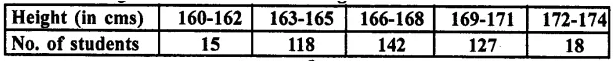Find the median height.
Solution:
Arranging the classes in exclusive form and then forming its cumulative frequency table as given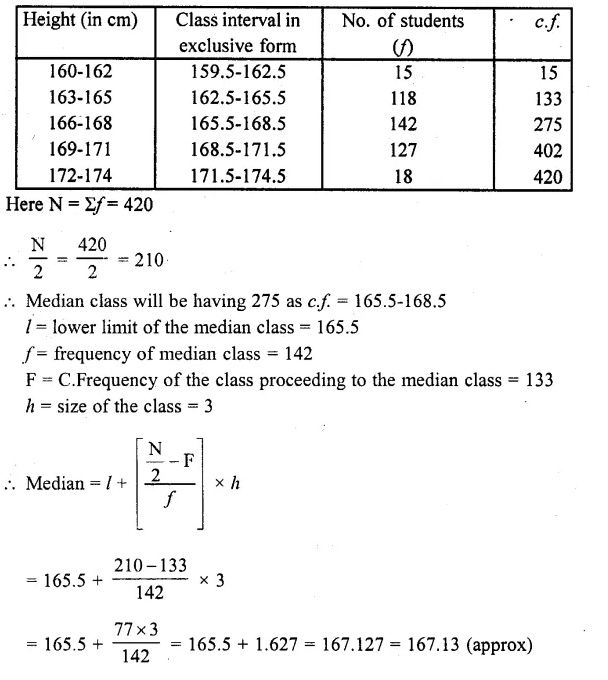Question 3.
Following is the distribution of I.Q. of 100 students. Find the median I.Q.Solution:
Arranging the classes in exclusive form and then forming it in cumulative frequency table as shown below :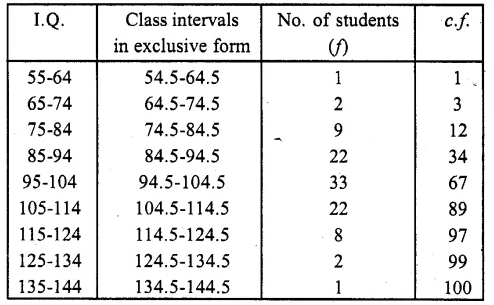Here N = 100
∴ $$\frac { N }{ 2 }$$ = $$\frac { 100 }{ 2 }$$ = 50
∴ Corresponding median class is = 94.5-104.5
l = 94.5, f= 33, F = 34 and h = 10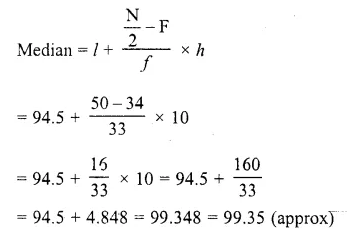Question 4.
Calculate the median from the following data :Solution:
Writing the given data in cumulative frequency table as shown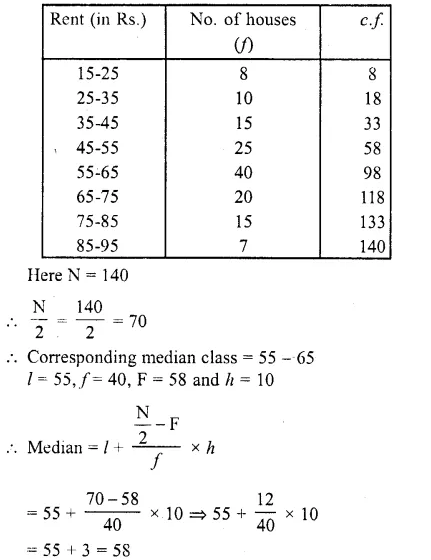Question 5.
Calculate the median from the following data :Solution: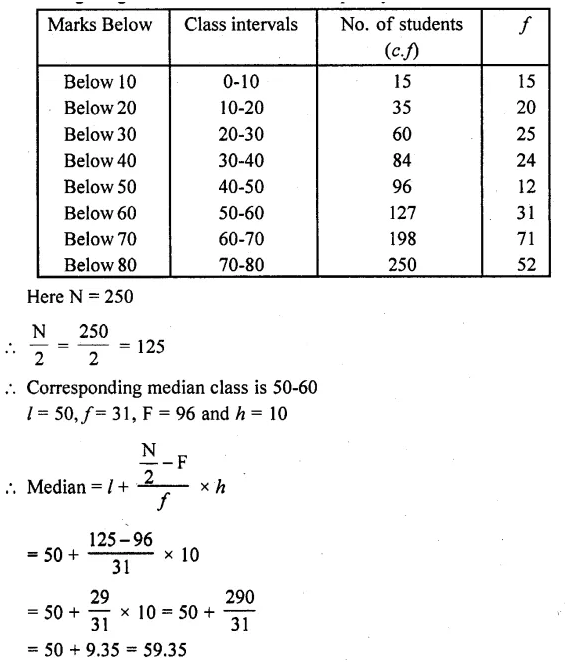Question 6.
Calculate the missing frequency from the following distribution, it being given that the median of the distribution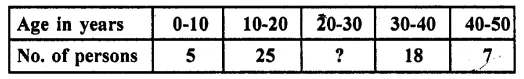Solution:
Median = 24, let p be the missing frequency.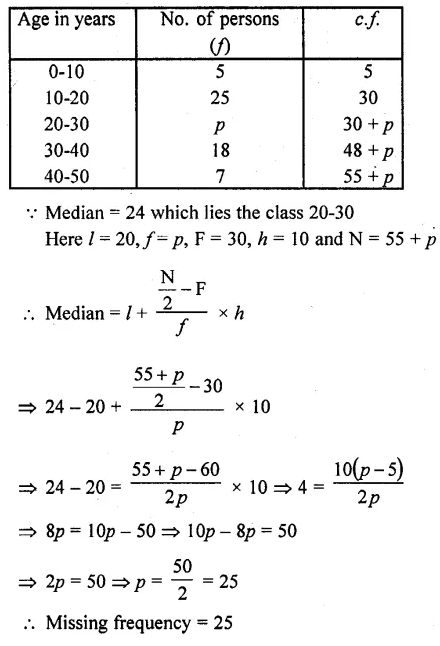Question 7.
The following table gives the frequency distribution of married women by age at marriage: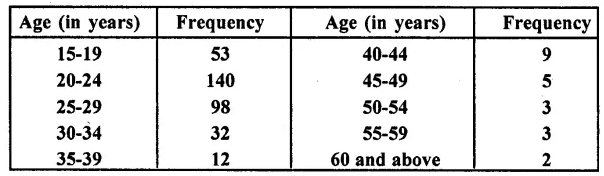Calculate the median and interpret the results.
Solution:
Writing classes in exclusive form,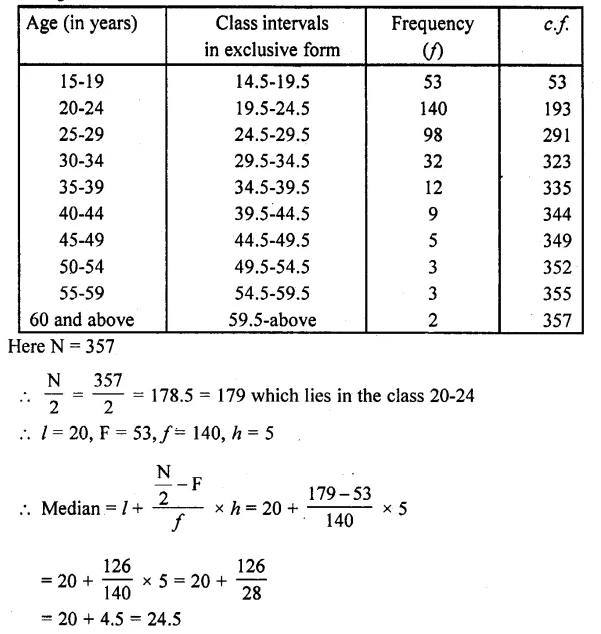Question 8.
The following table gives the distribution of the life time of 400 neon lamps :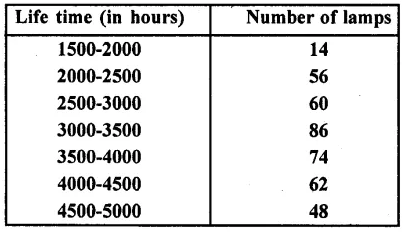Find the median life.
Solution: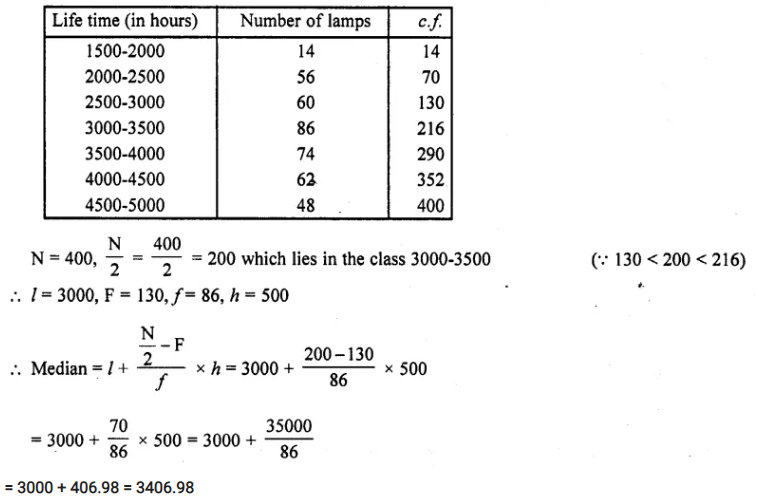= 3000 + 406.98 = 3406.98

Question 9.
The distribution below gives the weight of 30 students in a class. Find the median weight of students :Solution:
Here  N =30 ,$$\frac { N }{ 2 }$$ = $$\frac { 30 }{ 2 }$$ which  lies in the class 55 – 60 ( ∵ 13 < 15 < 19)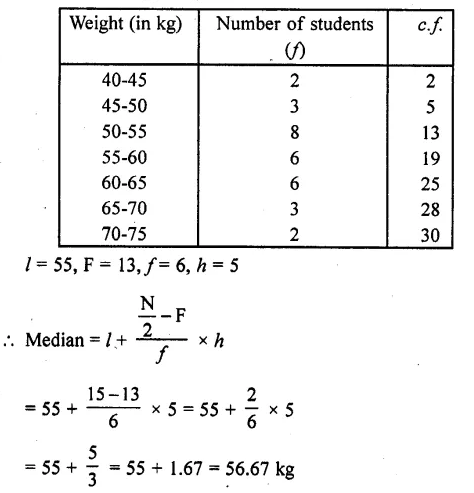Question 10.
Find the missing frequencies and the median for the following distribution if the mean is 1.46.Solution:
Mean = 1.46, N= 200
Let p1 and p2 be the missing frequencies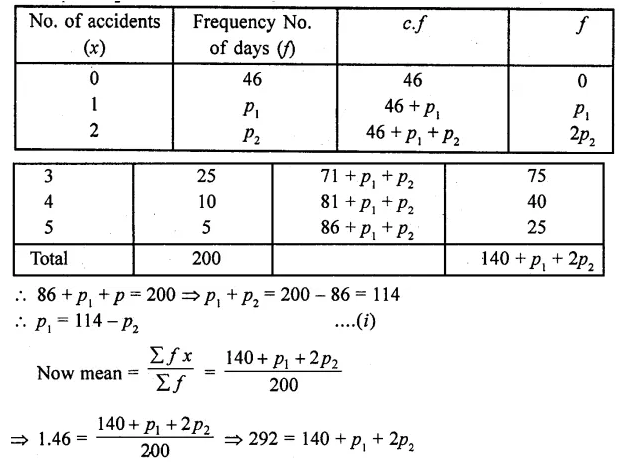⇒ p1+ 2p2= 292 – 140 = 152
114-p2 + 2p2= 152
⇒ p2 = 152- 114 = 38
∴ p1= 114-p2= 114-38 = 76
Hence missing frequencies are 76 and 38
Median = Here $$\frac { N }{ 2 }$$ = $$\frac { 200 }{ 2 }$$ = 100
∴ cf of 2nd class is 46 + 76 = 122
∴ Median = 1

Question 11.
An incomplete distribution is given below :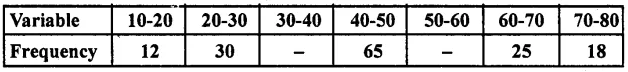You are given that the median value is 46 and the total number of items is 230.
(i) Using the median formula fill up missing frequencies.
(ii) Calculate the AM of the completed distribution.
Solution:
Let p1, and p2 be the missing frequencies
Median = 46 and N = 230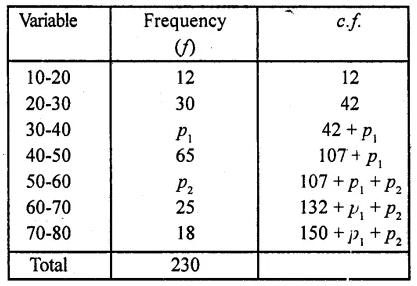∴ 150 + p1 + p2 = 230

⇒ p1+p2 = 230 – 150 = 80
∴ p2 = 80-p1 ….(i)
∵ Median = 46 which lies in the class 40-50
∴ I = 40, f= 65, F = 42 +p1, h = 10⇒ 39 = 73 – p1
⇒ p1 = 73 -39 = 34
∴ p2 – 80 – p1 = 80 – 34 = 46
Hence missing frequencies are 34, and 46
Mean Let assumed mean (A) = 45= 45 + 0.8695
= 45 + 0.87
= 45.87

Question 12.
If the median of the following frequency distribution is 28.5 find the missing frequencies: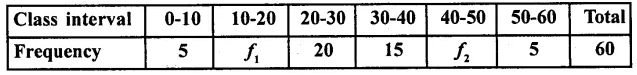Solution:
Mean = 28.5 , N = 6017 = 25 -f1
⇒ f1= 25 -17 = 8
and f2 = 15-f1 = 15-8 = 7
Hence missing frequencies are 8 and 7

Question 13.
The median of the following data is 525. Find the missing frequency, if it is given that there are 100 observations in the data:Solution:
Median = 525, N = 100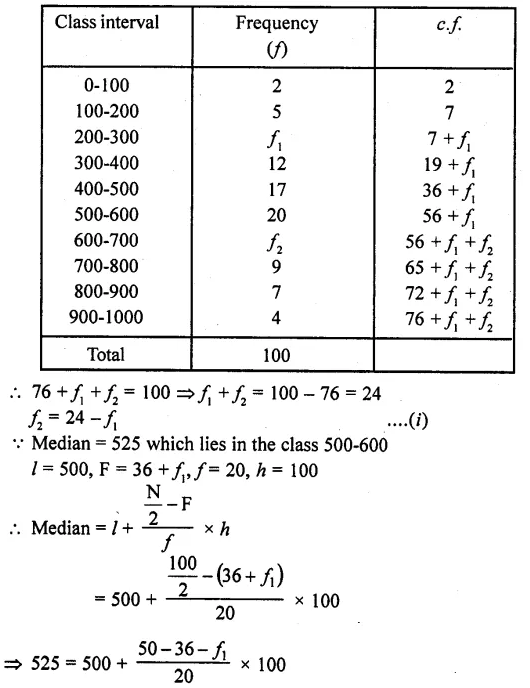⇒ 525 – 500 = (14 -f1) x 5
⇒ 25 = 70- 5f1
⇒ 5f1 = 70 – 25 = 45
⇒ f1 = $$\frac { 45 }{ 5 }$$ = 9
and f2 = 24 – f1 = 24 – 9 = 15
Hence f1 = 9, f2 = 15

Question 14.
If the median of the following data is 32.5, find the missing frequencies.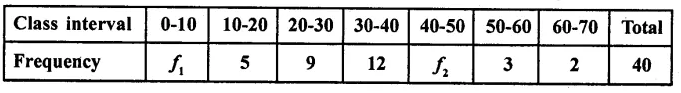Solution:
Mean = 32.5 and N= 40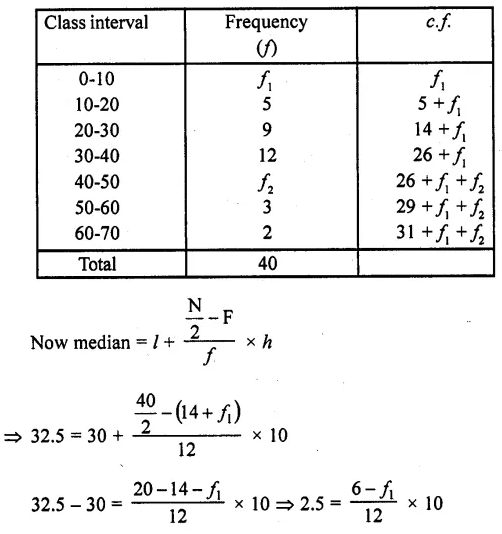⇒ 2.5 x 12 = 60 – 10f1
⇒ 30 = 60 – 10f1
⇒ 10f1 = 60-30 = 30
⇒ f1 = $$\frac { 30 }{ 10 }$$ =3
∴ f2 = 9 – f1 = 9-3 = 6
Hence f1 = 3, f2= 6

Question 15.
Compute the median for each of the following data: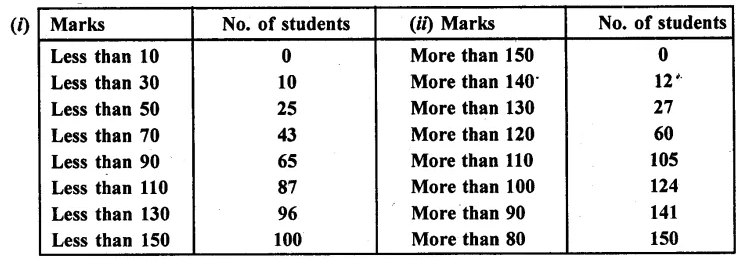Solution: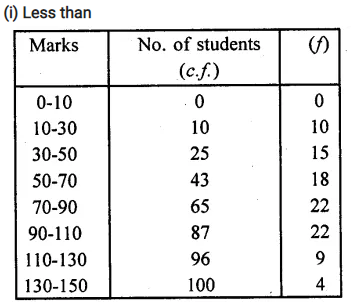Here N= 100=
∴ $$\frac { N }{ 2 }$$ = $$\frac { 100 }{ 2}$$ = 50 which lies in the class 70-90 (∵ 50 < 65 and > 43)
∴ l = 70, F =43 , f = 22 ,h = 20(ii) Greater thanN = 150, $$\frac { N }{ 2 }$$ = $$\frac { 150 }{ 2 }$$ = 75 which lies in the class 110-120 (∵ 75 > 105 and 75 > 60)
∴ l = 110, F = 60 , f=45, h= 10Question 16.
A survey regarding the height (in cm) of 51 girls of class X of a school was conducted and
the following data was obtained.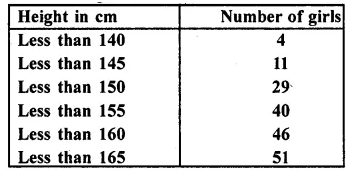Find the median height.
Solution: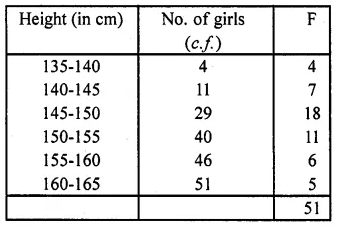Here $$\frac { N }{ 2 }$$ = $$\frac { 51 }{ 2 }$$ = 25.5 or 26 which lies in the class 145-150
l= 145, F= 11, f= 18, h= 5= 145 + 4.03 = 149.03

Question 17.
A life insurance agent found the following data for distribution of ages of 100 policy holders. Calculate the median age, if policies are only given to persons having age 18 years onward but less than 60 years.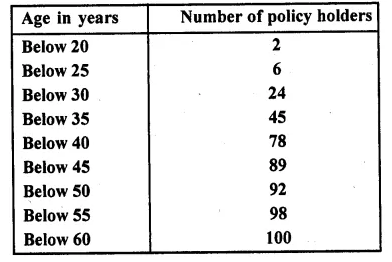Solution: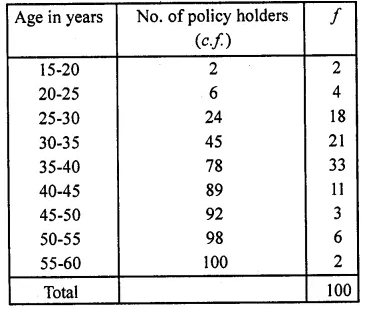Here N = 100, $$\frac { N }{ 2 }$$ = $$\frac { 100 }{ 2 }$$ = 50 which lies in the class 35-40 ( ∵ 45 > 50> 78)
l = 35, F = 45, f= 33, h = 5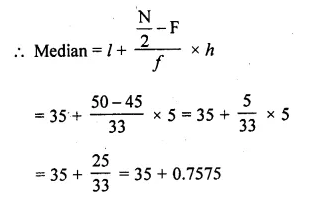= 35 + 0.76 = 35.76

Question 18.
The lengths of 40 leaves of a plant are measured correct to the nearest millimetre, and the data obtained is represented in the following table:Find the mean length of leaf.
Solution: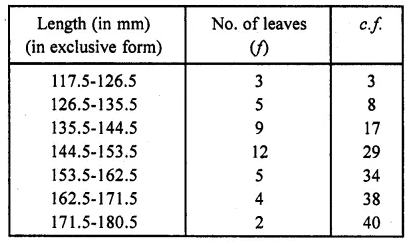N = 40, $$\frac { N }{ 2 }$$ = $$\frac { 40 }{ 2 }$$ = 20 which lies in the class 144.5-153.5 as 17 < 20 < 29
∴ l= 144.5, F= 17, f= 12, h = 9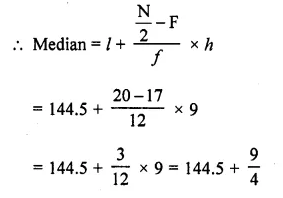= 144.5 + 2.25 = 146.75

Question 19.
An incomplete distribution is given as follows :You are given that the median value is 35 and the sum of all the frequencies is 170. Using the median formula, fill up the missing frequencies.
Solution:
Median = 25 and ∑f= N = 170
Let p1 and p2 be two missing frequencies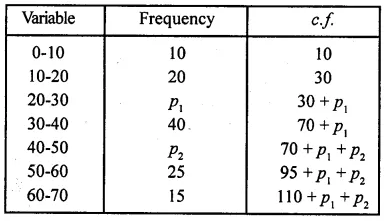∴ 110 + p1 +p2 = 170
⇒ p1+ p2 = 170 – 110 = 60
Here N = 170, $$\frac { N }{ 2 }$$ = $$\frac { 170 }{ 2 }$$ = 85
∴ Median = 35 which lies in the class 30-40
Here l = 30, f= 40, F = 30 + p1 and h = 10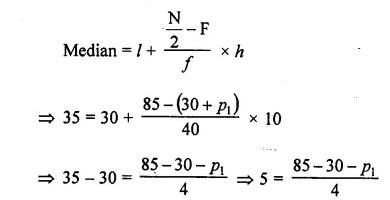20 = 55 – p1
⇒ p1 = 55 – 20 = 35
But p1+ p2 = 60
∴ p2 = 60 -p1 = 60 – 35 = 25
Hence missing frequencies are 35 and 25

Question 20.
The median of the distribution given below is 14.4. Find the values of x and y, if the total frequency is 20.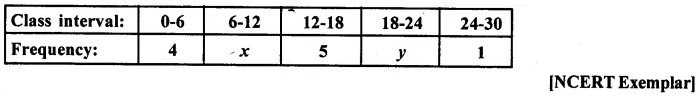Solution:It is given that n = 20
So, 10 + x + y – 20, i.e., x+y= 10 …(i)
It is also given that median = 14.4
Which lies in the class interval 12-18
So, l = 12, f= 5, cf = 4 + x, h = 6
Using the formula,Question 21.
The median of the following data is SO. Find the values of p and q, if the sum of all the frequencies is 90.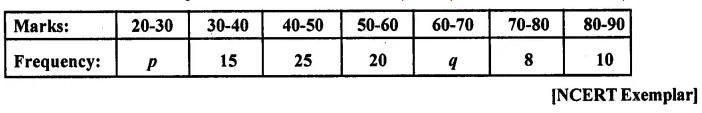Solution:Given, N = 90
∴ $$\frac { N }{ 2 }$$ = $$\frac { 90 }{ 2 }$$ = 45
Which lies in the interval 50-60
Lower limit, l = 50, f= 20, cf= 40 + p, h = 10∴ P = 5
Also, 78 +p + q = 90
⇒ 78 + 5 + q = 90
⇒ q = 90-83
∴ q = 7

Hope given RD Sharma Class 10 Solutions Chapter 15 Statistics Ex 15.4 are helpful to complete your math homework.

If you have any doubts, please comment below. Learn Insta try to provide online math tutoring for you.